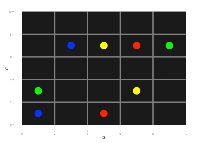## Wednesday, July 3, 2013

### Using R and Integer Programming to find solutions to FlowFree game boards

#### Using R and Integer Programming to find solutions to FlowFree game boards

What is FlowFree?
A popular game (iOS/Android) on a square board with simple rules. As the website states: Connect matching colors with pipes to create a flow. Pair all colors, and cover the entire board to solve each puzzle in Flow Free.

If you play a few games, you will be able to follow this post better. The games get progressively harder as you increase the board size.

The solutions to the various levels are available on the web, but it is fun to use R (and the lpSolveAPI package) to come up with solutions ourselves.

So let's say that the board is made up on n "cells" (small squares) on each side. A pipe can enter a cell from the center of any side. So each cell has 4 edges.

Figure: A cell with 4 edges meeting at the center

Terminal cells - Those cells where a colored-strand begins or ends. (Only one edge can be on in that cell.)

The 'rules' that we need to incorporate into our IP model
* The lines cannot cross.
* All the cells (squares) must be covered
* The colored lines must begin and end where they are shown

#### Decision variables

The problem comes down to deciding which edges to turn on, and which ones to leave off. An edge has a color and a cell. X_cke is 1 if edge e of color k in cell c is valid. 0 otherwise.

#### The constraints in English

1. Cell Cover Constraints: Every cell has to have 2 edges that turned on. (One to come in, one to exit)
1. Special case: Terminal cells have only one edge that can be 1. All other edges are 0.
2. An Edge can have only one color (LimitEdge constraints)
3. Horizontal Connectivity - If two cells are horizontal neighbors (side by side), then the value of the east edge of the left cell must be the same as the west edge of the cell on the right.
4. Vertical Connectivity - If two cells are vertical neighbors (one on top of the other), then the value of the downward edge of the top cell must be the same as the upward edge of the bottom cell
5. Boundary Edges - Certain edges for the cells in the boundary of the board are made zero. (For example, in the 4 corner cells, at most 2 edges can be 1.)
6. Single color in Terminal Cells - Each terminal cell has a pre-determined color given to us as input, so we can set of the edge varibles of every other color to be zero.
7.  Same color per cell & Pick 1 constraints. We set 0/1 variables and make sure that if a color is one inside a cell, ONLY that color edges are allowed in that cell.  (These are dense constraints and can make solution times explode for larger grids.)

All of the work of  creating the matrix, the right hand side are done by 3 functions - init(), populate.Amatrix() and defineIP(). [See github for the code] Now, let's initialize an empty data frame, based on number of constraints and variables that the IP will have.

#### Getting the problem ready for LPSolve

After using lpSolveAPI's solve() function, we plot the solution using ggplot2 to recreate the board and show the pipes in them.

### Let's try one problem:

>plotProb(terminal.cells, colorpalette)

###Here are the 8 terminal cells for this problem:
> terminal.cells
X Y color palette tcell
1 1 2     1   green     6
2 5 4     1   green    20
3 1 1     2    blue     1
4 2 4     2    blue    17
5 3 4     3  yellow    18
6 4 2     3  yellow     9
7 3 1     4     red     3
8 4 4     4     red    19

We create the linear program, and solve it using lpSolve

Let's see how many edges have non-zero values:
> lpff <- make.lp(nrow=n.row, ncol=n.col)
> defineIP()
> lpff
Model name:
a linear program with 500 decision variables and 458 constraints
> solve(lpff)
> sol <- get.variables(lpff)
> sum(unlist(sol[1:num.edges]))
 42
> plotSol(terminal.cells, colorpalette)

produces the solution:

And here's the solution when I tried an 8x8 (Level 17) grid:

Note: Because of same-color and pick-1 constraints, this problem explodes in size as n (the size of the grid) increases. There are however, ways to address it, using relaxation.

Future Improvements: The addition of the pick-1 and the same-color constraints spoil the structure of the A-matrix and increase the solution time. These can be made optional, in which case the solution will require manual intervention.